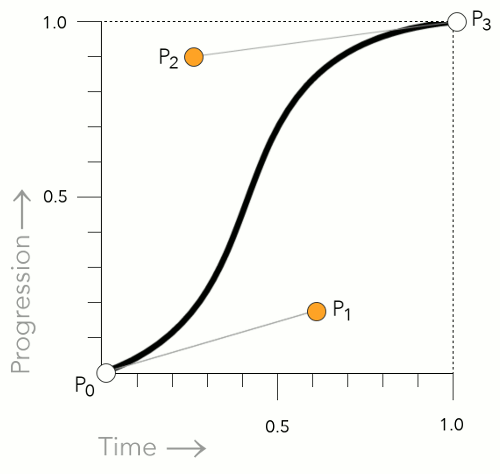# CSS cubic-bezier() Function

The `cubic-bezier()` function is used in CSS transitions to create a custom cubic Bézier curve.

The `cubic-bezier()` function can be used with the `transition-timing-function` property to control how a transition will change speed over its duration. This property accepts an easing function which describes how the intermediate values used during a transition will be calculated.

The `cubic-bezier()` function is one of the many possible easing functions that you can provide as a value for the `transition-timing-function` property.

Some of the other easing functions include `ease-in`, `ease-out`, `linear` etc. These use preset curves to determine how the transition progresses. However, the `cubic-bezier()` function can be used to provide your own custom curve.

## How to use the `cubic-bezier()` Function

A cubic Bézier curve is defined by four control points, P0, P1, P2, and P3 as shown in the following diagram.A cubic Bézier curve showing the Bézier timing function control points.

The P0 and P3 control points are always set to (0,0) and (1,1). In other words, they don't move.

However, P1 and P2 can be moved with the `cubic-bezier()` function. You can specify the location of these two control points by using an x and y value. Like this: `x1, y1, x2, y2`.

You specify these control points with number values. So an example might look like this:

This example sets the x and y values as follows:

## Working Example

Here's a working example of how the `cubic-bezier()` function works. Hover over the `ease` element, then the `cubic-bezier()` element to see how a different BÃ©zier curve changes the transition.

Notice in this case, the y2 value is higher than `1`. This results in a kind of "bouncing" effect, where the transition zooms past its end point, then returns back to it. Almost like a rubber band being stretched then returning to its normal state. This is perfectly acceptable. Both y values can exceed the [0,1] range, however, the x values must be within the [0,1] range.

You might also notice that this example uses the `transition` shorthand property, which allows us to set multiple properties at once (including the `transition-timing-function` property).

## Comparison with other Timing Functions

As mentioned, some of the other timing functions use preset curves to determine the transition's progress. Here are the values they use:

`ease`
Equivalent to `cubic-bezier(0.25, 0.1, 0.25, 1)`.
`linear`
This function is equivalent to `cubic-bezier(0, 0, 1, 1)`.
`ease-in`
This function is equivalent to `cubic-bezier(0.42, 0, 1, 1)`.
`ease-out`
This function is equivalent to `cubic-bezier(0, 0, 0.58, 1)`.
`ease-in-out`
This function is equivalent to ```cubic-bezier(0.42, 0, 0.58, 1)```.

So in other words, the following two declarations are equivalent:

## Official Syntax

The official syntax of the `cubic-bezier()` function is as follows:

As mentioned, the four values listed in the syntax specify points P1 and P2 of the curve as `(x1, y1, x2, y2)`. Like this

Both x values must be number values in the range [0, 1]. The y values can exceed this range.

## CSS Specifications

• The `cubic-bezier()` function is defined in CSS Transitions (W3C Working Draft 19 November 2013)

## Browser Support

The following table provided by Caniuse.com shows the level of browser support for this feature.